SSC  >  SSC CPO Mock Test (GI & Reas) 2

# SSC CPO Mock Test (GI & Reas) 2

Test Description

## 50 Questions MCQ Test SSC CPO & Constable - Mock Tests & Previous Year Papers | SSC CPO Mock Test (GI & Reas) 2

SSC CPO Mock Test (GI & Reas) 2 for SSC 2023 is part of SSC CPO & Constable - Mock Tests & Previous Year Papers preparation. The SSC CPO Mock Test (GI & Reas) 2 questions and answers have been prepared according to the SSC exam syllabus.The SSC CPO Mock Test (GI & Reas) 2 MCQs are made for SSC 2023 Exam. Find important definitions, questions, notes, meanings, examples, exercises, MCQs and online tests for SSC CPO Mock Test (GI & Reas) 2 below.
Solutions of SSC CPO Mock Test (GI & Reas) 2 questions in English are available as part of our SSC CPO & Constable - Mock Tests & Previous Year Papers for SSC & SSC CPO Mock Test (GI & Reas) 2 solutions in Hindi for SSC CPO & Constable - Mock Tests & Previous Year Papers course. Download more important topics, notes, lectures and mock test series for SSC Exam by signing up for free. Attempt SSC CPO Mock Test (GI & Reas) 2 | 50 questions in 30 minutes | Mock test for SSC preparation | Free important questions MCQ to study SSC CPO & Constable - Mock Tests & Previous Year Papers for SSC Exam | Download free PDF with solutions
 1 Crore+ students have signed up on EduRev. Have you?
SSC CPO Mock Test (GI & Reas) 2 - Question 1

### Complete the analogous pair.BAC : DEF :: ? : NOP

SSC CPO Mock Test (GI & Reas) 2 - Question 2

### Find out which word or name will be in the middle after the words or names are arranged in the alphabetical order.

SSC CPO Mock Test (GI & Reas) 2 - Question 3

### Re-arrange the given words in alphabetical order and tick the one that comes in the middle.

SSC CPO Mock Test (GI & Reas) 2 - Question 4
Complete the analogous pair.
Depth : Fathom :: Speed : ?
SSC CPO Mock Test (GI & Reas) 2 - Question 5
Choose the word which is least like the other words in the group .
Detailed Solution for SSC CPO Mock Test (GI & Reas) 2 - Question 5 All except Stone are obtained directly or indirectly from trees.
SSC CPO Mock Test (GI & Reas) 2 - Question 6
Choose the group of letters which is different from others.
SSC CPO Mock Test (GI & Reas) 2 - Question 7
Complete the analogous pair.
Good : Bad : : Virtue : ?
SSC CPO Mock Test (GI & Reas) 2 - Question 8
Choose the word which is least like the other words in the group .
SSC CPO Mock Test (GI & Reas) 2 - Question 9
Complete the analogous pair.
Envelop : Envelope :: Float : ?
SSC CPO Mock Test (GI & Reas) 2 - Question 10
Complete the analogous pair.
Painting : Artist : : Symphony : ?
SSC CPO Mock Test (GI & Reas) 2 - Question 11
A policeman goes straight seven (7) kilometers eastwards, then turns right and goes straight three (3) kilometers and turns right again and goes straight ten (10) kilometers. In which direction is he from the starting point?
SSC CPO Mock Test (GI & Reas) 2 - Question 12
If the letters from T to Z are interchanged in opposite order by the letter A to G in such a way that A takes the position of T and So on, then which will be the third letter to the right of 18th letter from left?
SSC CPO Mock Test (GI & Reas) 2 - Question 13

After walking 6 km, I turned right and covered a distance of 2 km, then turned left and covered a distance of 10 km. In the end, I was moving towards the north. From which direction did I start my journey ?

Detailed Solution for SSC CPO Mock Test (GI & Reas) 2 - Question 13

Clearly, the route is as shown in the adjoining diagram. Thus, I started the journey from the South and moved northwards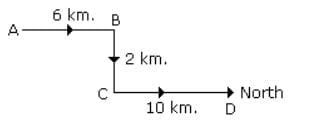SSC CPO Mock Test (GI & Reas) 2 - Question 14
Find the missing character.
Detailed Solution for SSC CPO Mock Test (GI & Reas) 2 - Question 14 In fig. (A), (915-364)=551.
In fig. (B), (789-543)=246.
$4$In fig. (C), missing number = (863-241)=622.
SSC CPO Mock Test (GI & Reas) 2 - Question 15
Group the following figures into three classes on the basis of identical properties.
Detailed Solution for SSC CPO Mock Test (GI & Reas) 2 - Question 15 1,4,7 are all (two-dimensional) quadrilaterals.
2,5,8 are all three-dimensional figures.
3,6,9 are all (two-dimensional) triangles.
SSC CPO Mock Test (GI & Reas) 2 - Question 16
Find the number of rectangles in the given figure.
Detailed Solution for SSC CPO Mock Test (GI & Reas) 2 - Question 16 The figure may be labelled as shown.

The simplest rectangles are ABQP, PQNO, BCDN, NDEM, MEFL, LFJK, FGHR and RHIJ i.e. 8 in number.
The rectangles composed of two components each are ABNO, BCEM, NDFL, MEJK and FGIJ i.e. 5 in number.
The rectangles composed of three components each are ACDO, BCFL, NDJK and LGIK i.e. 4 in number.
There is only one rectangle i.e. BCJK composed of four components.
Total number of rectangles in the figure = 8 + 5 + 4 + 1 = 18.
SSC CPO Mock Test (GI & Reas) 2 - Question 17
Find the missing character.
SSC CPO Mock Test (GI & Reas) 2 - Question 18
Choose the odd one out.
SSC CPO Mock Test (GI & Reas) 2 - Question 19
Choose the odd one out.
SSC CPO Mock Test (GI & Reas) 2 - Question 20
Find the missing character.
SSC CPO Mock Test (GI & Reas) 2 - Question 21
How many such letter-pairs are there in the word BONAFIDE having same no. of letters left between them as they have in the series?
Detailed Solution for SSC CPO Mock Test (GI & Reas) 2 - Question 21 Six letter-pairs of the word are :
N - O, B - F, A - D, D - F, D - E and A - E
SSC CPO Mock Test (GI & Reas) 2 - Question 22
If XY = 600, ABC = 6, then GO + DO will be equal to:
SSC CPO Mock Test (GI & Reas) 2 - Question 23
If MAGIC is coded as PXJFF then how will LEASH be coded?
Detailed Solution for SSC CPO Mock Test (GI & Reas) 2 - Question 23
SSC CPO Mock Test (GI & Reas) 2 - Question 24
Given interchanges : signs + and - numbers 8 and 10.
Which statement is true?
Detailed Solution for SSC CPO Mock Test (GI & Reas) 2 - Question 24 Option. A
8 - 10 + 6 = 12
if we interchange the no 8 and 10 and signs + and - we get
10 + 8 - 6 = 12
⇒ 18 - 6 = 12 (correct)
Option. B
10 - 8 + 7 = 4
if we interchange the no 8 and 10 and signs + and - we get
⇒ 8 + 10 - 7 = 4
⇒ 18 - 7 = 4
⇒ 11 ≠ 4 (not true)
Option. C
10 + 8 - 9 = 16
if we interchange the no 8 and 10 and signs + and - we get
8 - 10 + 9 = 16
⇒ 17 - 10 = 16
⇒ 7 ≠ 16 (not true)
Option. D
10 + 8 - 3 = 10
if we interchange the no 8 and 10 and signs + and - we get
8 - 10 + 3 = 10
⇒ 11 - 10 = 10
⇒ 1 ≠ 10 (not true)
SSC CPO Mock Test (GI & Reas) 2 - Question 25

Some equations are solved on the basis of certain system. Find out the correct answer for the unsolved equation on that basis
2 × 3 × 7 = 4949, 5 × 9 × 4 = 258116, 4 × 6 × 8 = ?

Detailed Solution for SSC CPO Mock Test (GI & Reas) 2 - Question 25

2 × 3 × 7
= (2)2 × (3)2 × (7)2
= 4949
5 × 9 × 4
= (5)2 × (9)2 × (4)2
= 258116
So, 4 × 6 × 8
= (4)2 × (6)2 × (8)2
= 163664

SSC CPO Mock Test (GI & Reas) 2 - Question 26

If 2 * 3 = 12, 3 * 4 = 20 and 4 * 5 = 30, then 2 * 6 is

Detailed Solution for SSC CPO Mock Test (GI & Reas) 2 - Question 26

The numbers on both sides of * are increased by one and then multiplied to get the answer. 2*6 = 3 × 7 =  21

SSC CPO Mock Test (GI & Reas) 2 - Question 27
Which of the following diagrams illustrate the relationship among Science, Maths and Biology?
Detailed Solution for SSC CPO Mock Test (GI & Reas) 2 - Question 27 (A) Biology is a branch of Science. Maths is different from both i.e.
SSC CPO Mock Test (GI & Reas) 2 - Question 28
Complete the analogous pair.
23:13::54: ?
SSC CPO Mock Test (GI & Reas) 2 - Question 29
Choose the odd one out.
SSC CPO Mock Test (GI & Reas) 2 - Question 30
Choose the odd one out.
SSC CPO Mock Test (GI & Reas) 2 - Question 31
Choose the one which is different from the rest:
SSC CPO Mock Test (GI & Reas) 2 - Question 32
In this letter series, some of the letters are missing. Choose the correct letter given below -
b _ abbc _ bbca _ bcabb _ ab
SSC CPO Mock Test (GI & Reas) 2 - Question 33
Complete the series.
7714, 7916, 8109?
SSC CPO Mock Test (GI & Reas) 2 - Question 34
In this letter series, some of the letters are missing. Choose the correct letter given below -
gfe _ ig _ eii _ fei _ gf _ ii
SSC CPO Mock Test (GI & Reas) 2 - Question 35
Which number would replace question mark in the series 7, 12, 19, ?, 39.
SSC CPO Mock Test (GI & Reas) 2 - Question 36
Find out from amongst the four alternatives as to how the pattern would appear when the transparent sheet is folded at the dotted line.
SSC CPO Mock Test (GI & Reas) 2 - Question 37
Find out the term in the number series which is wrong.
24, 27, 31, 33, 36
SSC CPO Mock Test (GI & Reas) 2 - Question 38
In this letter series, some of the letters are missing. Choose the correct letter given below -
_ bcc _ ac _ aabb _ ab _ cc
SSC CPO Mock Test (GI & Reas) 2 - Question 39
In each question a statement followed by two assumptions numbered I and II is given. Consider the statement and decide which of the given assumption is implicit.
Statement : The economic prosperity of any nation is dependent on the quality of its human resources.
Assumptions : I. It is possible to measure the quality of human resources of a nation.
II. Achieving economic prosperity is a cherished goal of every nation.
SSC CPO Mock Test (GI & Reas) 2 - Question 40
Below is given statement followed by three conclusions numbered I, II and III. You have to consider the statement and the following conclusions and decide which of the conclusions is follows in the statement :
Statements :a. Some blades are hammers.
b. Some hammers are knives.
c. Some knives are axes.
Conclusions:I. Some axes are hammers.
SSC CPO Mock Test (GI & Reas) 2 - Question 41
If 'red means 'white', 'white' means 'black', 'black' means 'yellow', 'yellow' means 'green' and 'green' means 'blue' and 'blue means 'indigo'; then which of the following will represent the colour of sunflower?
SSC CPO Mock Test (GI & Reas) 2 - Question 42
According to Ramanuj to birthday of her sister is after January 15 but before January 20. According to the father of Ramanuj, the birthday of Ramanuj's sister is after January 18 but before 22. What is the birthday of Ramanuj's sister?
SSC CPO Mock Test (GI & Reas) 2 - Question 43
3 out of 4 words are alike in some way and form a group. Which one does not belong to the group?
SSC CPO Mock Test (GI & Reas) 2 - Question 44
A word given in Capital letter is followed by four answer words. Out of these only one cannot be formed by using the letters of the given word. find out that word.
CONSTRUCTION
SSC CPO Mock Test (GI & Reas) 2 - Question 45

In the following questions, select a figure from amongst the four alternatives, which when placed in the blank space of fig. (X) would complete the pattern.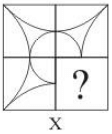SSC CPO Mock Test (GI & Reas) 2 - Question 46

In each question below is given a group of letters followed by four combinations of digits numbered (A), (B), (C) and (D). You have to find put which of the combinations correctly represents the group of letters based on the following coding system and mark the number of that combination as the answer. If none of the four combinations correctly represents the group of letters, mark (E) i.e. 'None of these' as the answer.
Letter : B L C X P D S K M E
Digits :  7 1  0  8 9  3 6   2   5  4

SXEKBDM

SSC CPO Mock Test (GI & Reas) 2 - Question 47

Study the following diagram.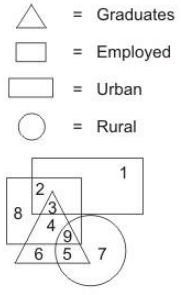Q. What does number 4 represent?

SSC CPO Mock Test (GI & Reas) 2 - Question 48

Select the correct mirror-image of the Figure (X) from amongst the given alternatives.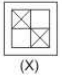SSC CPO Mock Test (GI & Reas) 2 - Question 49

Each of the following question consists of five problem figures marked 1,2,3,4,5 followed by answer figures marked A,B,C,D and E. Select figure from answer figures which will continue the same series as given in the problem figures.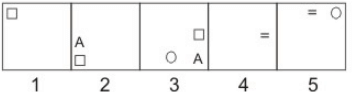Detailed Solution for SSC CPO Mock Test (GI & Reas) 2 - Question 49

The three new designs from half side back in the 1st three figures and the new design forms ahead 1/2 side ACW of the front side design. The same changing sequence continues in the next three figures.

SSC CPO Mock Test (GI & Reas) 2 - Question 50

In the questions, figure (X) is embedded in one of following figures. Find out the correct alternative.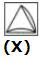## SSC CPO & Constable - Mock Tests & Previous Year Papers

1 docs|63 tests
 Use Code STAYHOME200 and get INR 200 additional OFF Use Coupon Code
Information about SSC CPO Mock Test (GI & Reas) 2 Page
In this test you can find the Exam questions for SSC CPO Mock Test (GI & Reas) 2 solved & explained in the simplest way possible. Besides giving Questions and answers for SSC CPO Mock Test (GI & Reas) 2, EduRev gives you an ample number of Online tests for practice

1 docs|63 tests